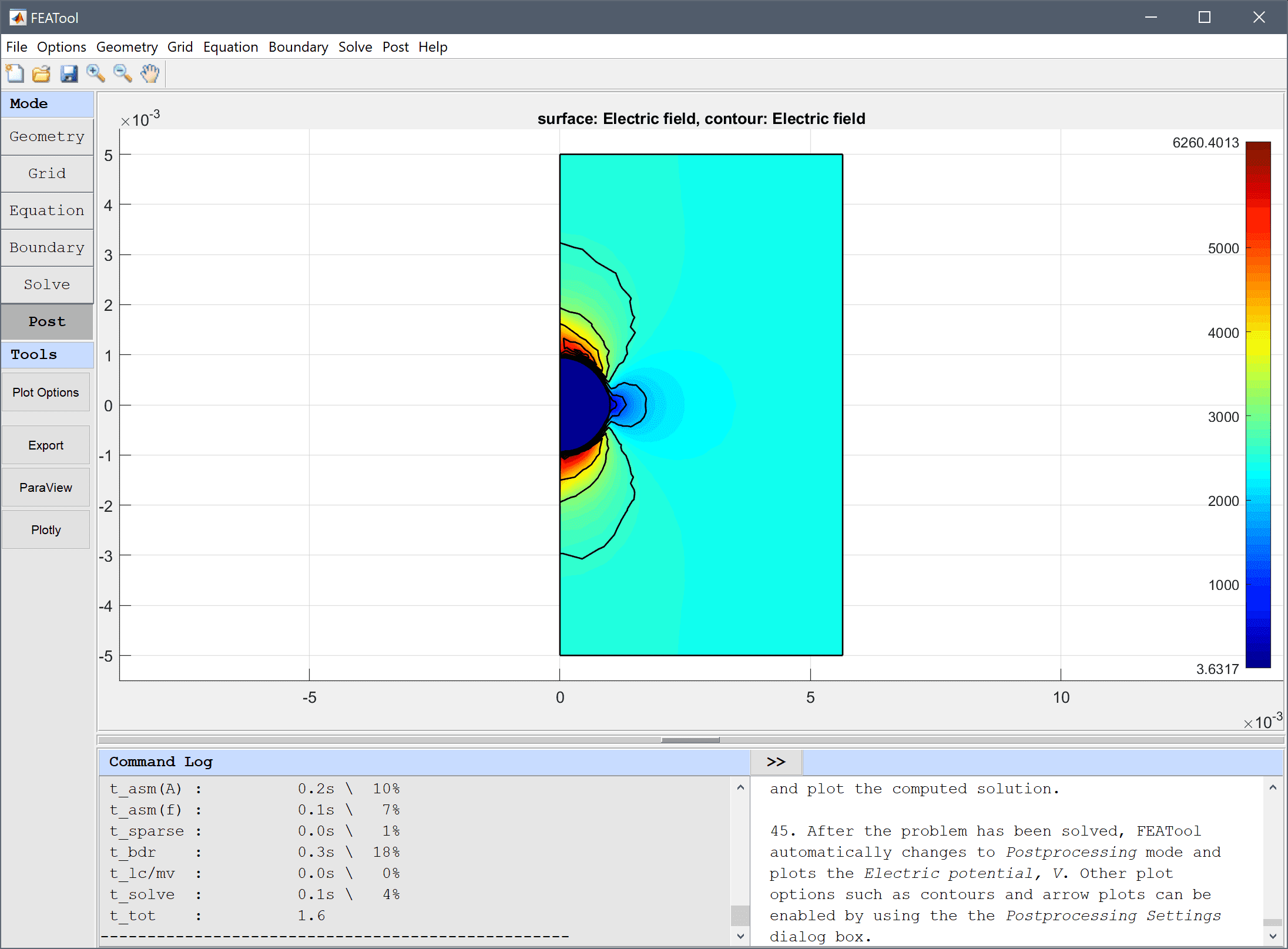# Conducting Sphere## Model Data

Type: electromagnetics

Physics Modes: electrostatics

Keywords: capacitor axisymmetry verification

This verification example considers a perfectly conducting sphere placed between two charged capacitor plates. An axisymmetric solution for the electrostatic potential is compared with a full 3D one, and verified against the analytical solution U(xi) = E0*(r3/xi2 - xi), where the electrical field is the potential difference divided by the distance between the plates Ei = dV/di.

In this example the conductive sphere is given a radius of 1 mm while the distance between the places is 1 cm. The area of the places is taken as 0.1 x 0.1 m and in axisymmetric coordinates a circle with equivalent area. The applied voltage is 20 V and dielectric permittivity of the medium ε = 5.

This model is available as an automated tutorial by selecting Model Examples and Tutorials… > Electromagnetics > Conducting Sphere from the File menu. Or alternatively, follow the step-by-step instructions linked below.